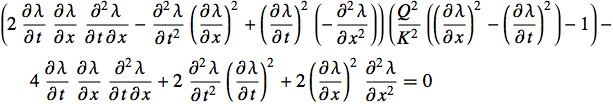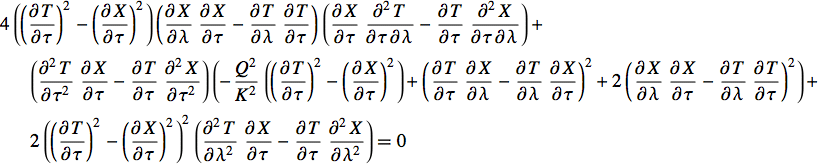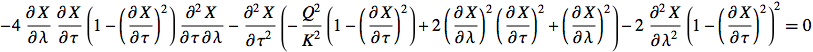# Relativistic Elasticity

## by Greg Egan

In thought experiments in special relativity, when a rocket engine pushes on the rear of a spacecraft, or a tether is dragged behind an accelerating craft, assuming that these extended objects respond to the forces on them instantly and without deformation can lead to confusion and apparent paradoxes. The idealisation of a rigid body, which can often be useful in Newtonian physics, is generally no longer applicable, since it corresponds to an assumption of an infinite speed of sound within the body, in violation of relativistic causality.

In many cases, a qualitative understanding that perfectly rigid motion will be the exception rather than the rule in special relativity is enough to avoid the pitfalls. At other times, though, it can be useful to have a simple, quantitative model that deals with elastic deformation in a way that is consistent with special relativity.

The purpose of this page is to describe such a model of relativistic elasticity. We will restrict ourselves to the simplest possible situation: one-dimensional hyperelastic bodies. By “hyperelastic”, we mean the body will obey Hooke’s law, which states that any change in length of a portion of the body (compared to its unstretched, or “relaxed” length) will be proportional to the tension to which it is subjected. For any real material, Hooke’s law is just an approximation which holds over a limited range of tension and compression. However, for the sake of producing a (reasonably) mathematically tractable model, we will treat it as an exact law which holds up to the point where the material breaks.

We will require that the speed of sound in the material being modelled is less than the speed of light, which puts a finite limit on its stiffness. We will also assume that the material must break before the tension in it becomes greater than its energy density, which would violate the weak energy condition. (For all known matter fields the tension is less than the energy density, so certainly any normal material is expected to obey the weak energy condition.)

Since we are dealing with only one spatial dimension, we can label each element of our elastic body with a single number, λ, which we define as the distance from some chosen point on the body when it’s in its relaxed state. Then, when the body is undergoing some arbitrary motion, the totality of the world lines for all of its elements will occupy a certain region of spacetime. In that region, any event will lie on exactly one world line, so we can think of the world lines’ labels, λ, as giving us a scalar-valued function on spacetime. The world lines are then the contours of that scalar function. One approach we will consider will lead us to a partial differential equation for λ; the other approach will be to treat the world lines as a family of curves in spacetime, and to find a PDE for the functions describing those curves. Both approaches are equivalent, of course, but which is easiest to apply will depend on the nature of the particular problem being analysed.

As well as the scalar function λ, our spacetime region will have a vector field u which gives the 4-velocity of each element of the elastic body. We will also define a unit spacelike vector field w, orthogonal to u; for convenience, we would normally choose the sign of w so that it has a positive spatial coordinate in any inertial frames we’re using. Both u and w are determined by λ, but for now we will simply take them as given.

We can measure how much the elastic body has been stretched or compressed at any point by defining a scalar n:

n = |∇λ|

This is the magnitude of the gradient of λ, so it tells us how tightly spaced the contours of λ are. When the body is relaxed, n=1; when it is stretched, n<1; when it is compressed, n>1. To put it another way, a piece of material of unit length in its relaxed state will have an actual length of 1/n.

The quantity n will determine the elastic potential energy density in the material, and from that the pressure can be derived. Given our assumption of hyperelasticity, the appropriate formulas are:

ε = (k/2) (1 – 1/n)2
p = k (1 – 1/n)

Here k is the 1-dimensional Young’s modulus for the material, which determines its stiffness. The elastic potential energy density, ε, is given here per unit of unstretched length, and so it must be multiplied by n to give an actual energy density. (Since 1/n is the actual length of an element of unit unstretched length, the pressure is found by making the substitution n → 1/s, taking the opposite of the derivative, p = –dε/ds, and then substituting back for n.)

It’s important to note that this model is not well-behaved for large compressions, since it allows an extended object to be squashed down to zero length by a finite pressure (as n → ∞, pk). For small compressions it will give reasonable results, but for large compressions a more sophisticated model would be required, in which the potential energy was modified to provide a more realistic response.

If we are going to respect the weak energy condition, then we require a limit on the tension, –p:

p < n (ρ + ε)

where ρ is the rest mass density of the material in its relaxed state. This leads to a limit on the “stretch factor”, s:

s = 1/n < Q / K

where we have introduced the parameters:

K2 = k/(2 ρ)
Q2 = 1 + K2

Knowing the energy density and pressure in the material, we can find its stress-energy tensor, T:

T = n (ρ+ε) uu + p ww

In special relativity, conservation of momentum is satisfied by requiring that the divergence of this tensor is zero. The divergence of a tensor is a vector, and we can obtain a scalar equation by taking the Lorentzian dot product of the divergence with the vector field w:

w · (div T) = a (n(ρ+ε) + p) + ∇wp = 0

Here, a is the proper acceleration of the elements of the body, the magnitude of the vector a=∇uu. We will not make use of the dot product of the divergence with u, because this turns out to be identically zero.

Physically, we can interpret this equation as something quite similar to the Newtonian equation F = ma, applied to an element of the elastic body of unit spatial length (actual length, not unstretched length). The gradient of the pressure, ∇wp, is the opposite of the force on the element, and n(ρ+ε) is the mass, including the potential energy. The extra term, a p, is necessary to conserve momentum, and arises because the momentum flow associated with the pressure will be changing direction in spacetime when the element is accelerating, “rotating” it into a momentum density.

We can express the pressure and energy density in terms of n, and rearrange the equation to obtain:

a n [(Q2/K2) n2 – 1] + 2 ∇wn = 0

If we take the approach of seeking to express everything in terms of λ, we can start by noting that:

w = vector field corresponding to (∇ λ) / n

Here ∇ λ is a covector or 1-form field, and what we mean by equating w to the corresponding vector field, normalised by n, is that we “raise an index” using the Minkowski metric. Geometrically, we’re just saying that w is orthogonal to the contours of λ. In terms of coordinates {t,x} in some inertial frame:

n = √[(∂xλ)2 – (∂tλ)2]
w = [(–∂tλ) ∂t + (∂xλ) ∂x] / n
u = [(∂xλ) ∂t – (∂tλ) ∂x] / n

We won’t give the detailed derivation of the acceleration a and of ∇wn, but the final equation that is obtained, a PDE for λ, is:We obtained one family of exact solutions to this equation in the page on the Rindler Horizon:

λ(s)/s1 = (K/Q3) [log[Q √(s/s1) + √(Q2s/s1 – 1)] – log[Q + K] + Q [√(s/s1 (Q2s/s1 – 1)) – K]]
where s = √(x2t2)

These solutions are invariant under boosts (since boosts leave s invariant). They describe objects that are static in a Rindler frame, and which have zero tension at s=s1, i.e. suspended strings with their bottom ends at s1. (As noted, our hyperelastic model is not well-behaved for high compression, so it would not be safe to extend these solutions to the case of columns under pressure near a Rindler horizon.)

The alternative approach is to consider the world line of each element to be one of a family of curves in spacetime. We label each curve by λ, and then parameterise it by a parameter τ; this can be chosen to be the proper time along the curve, if that’s convenient, but there’s no reason not to use other choices of parameter, and they will often be much simpler. In an inertial coordinate system, we describe the world lines by means of two real-valued functions:

(t,x) = (T(τ,λ), X(τ,λ))

All the necessary quantities can then be expressed in terms of these two functions, and the resulting equation is:In order to eliminate T, leaving a single PDE for X, we need to make an explicit choice for the parameter τ. For example, if we decide to set τ equal to the time coordinate t, we obtain:As an example of a simple application for this equation, let’s consider the case where we have infinitesimal deformations of an elastic body. If we set:

X(τ,λ) = λ + A Z(τ,λ)

and then discard terms that are higher than first order in A, the result is:

t,tZ = 2 K2λ,λZ

This is just the linear wave equation, with a speed of sound equal to K√2. So for infinitesimal deformations, there is no difference between relativistic and classical elasticity. This also makes it clear that we need to impose the condition:

K < 1/√2

in order that the speed of sound does not exceed the speed of light. We can generalise this to the case of an infinite elastic string under almost constant tension. If we set:

X(τ,λ) = λ / n + A Z(τ,λ)

and then discard terms that are higher than first order in A, the result is:

t,tZ = 2 K2 n2 / (Q2 n2K2) ∂λ,λZ

Again this is just the linear wave equation; if we take account of the near-constant stretch factor of s=1/n that converts λ to X, this implies a speed of sound equal to K√[2 / (Q2 n2K2)]. So to ensure a speed of sound less than the speed of light, we need to impose the condition:

s = 1/n < Q / (√3 K)

which limits the factor by which the material can be stretched to just 1/√3 times the ceiling imposed by the weak energy condition. Note that as K approaches 1/√2, the value at which the speed of sound equals the speed of light even in the relaxed material, this upper bound on the stretch factor approaches 1.

Other choices of parameter along the world lines can be convenient, depending on the boundary conditions. For the numerical results in the page on the Rindler Horizon for a string being unreeled at a constant rate, T was offset by the t coordinate of the point of unreeling:

T(τ, λ) = s2 sinh(λ / (R s2)) + τ

An approximate solution was found by setting X equal to a polynomial P in λ and τ, plus an initial offset equal to the x-coordinate of the point of unreeling:

X(τ, λ) = s2 cosh(λ / (R s2)) + P(τ, λ)

This made the boundary condition describing the way the string was unreeled very simple:

P(0, λ) = 0

“Relativistic Elastodynamics” by Michael Wernig-Pichler is a wonderful PhD thesis which treats elasticity in the full general-relativistic context.

### Notes

 One simple model that is reasonably well-behaved for extreme compressions (but not for large tensions) is:

ε = k (log n + 1/n – 1)
p = k (n–1)

The log term means the resulting PDE is not only non-linear but non-polynomial. (Amazingly enough, the boost-invariant case can be explicitly solved, at least in terms of integrals of exp(–t)/t as well as standard elementary functions.) To first order these formulas agree with the hyperelastic model, but while the hyperelastic model becomes absurd for large compressions, this model is equally absurd for large tensions, since it allows an object to become infinitely stretched by a finite tension (as n → 0, p → –k).Science Notes / Relativistic Elasticity / created Monday, 19 February 2007 / revised Friday, 20 July 2007